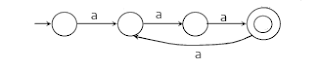Type Here to Get Search Results !

# Formal Languages And Automation Theory MCQs With Answers - Set IV

0

Formal Languages And Automation Theory MCQs

Set IV

Q1. Which of the given are correct?

a) Moore machine has 6-tuples

b) Mealy machine has 6-tuples

c) Both Mealy and Moore has 6-tuples

d) None of the mentioned

Answer is c) Both Mealy and Moore has 6-tuples
Explanation : The six tuples are (Q, ∑, O, δ, X, q0)
It can be described by a 6 tuple (Q, ∑, O, δ, X, q0) where −
Q is a finite set of states.
∑ is a finite set of symbols called the input alphabet.
O is a finite set of symbols called the output alphabet.
δ is the input transition function
X is the output transition function
q0 is the initial state from where any input is processed (q0 ∈ Q).

Q2. Which of the following does not belong to input alphabet if S={a, b}* for any language?

a) a

b) b

c) e

d) none of the above

Explanation : The automaton may be allowed to change its state without reading the input symbol using epsilon but this does not mean that epsilon has become an input symbol. On the contrary, one assumes that the symbol epsilon does not belong to any alphabet.

Q3. Design a NFA for the language:
L: {an| n is even or divisible by 3}
Which of the following methods can be used to simulate the same.

a) e-NFA

b) Power Construction Method

c) Both (a) and (b)

d) None of the mentioned

Answer is c) Both (a) and (b)
Explanation : It is more convenient to simulate a machine using e-NFA else the method of Power Construction is used from the union-closure of DFA’s.

Q4. State true or false:
Statement: Both NFA and e-NFA recognize exactly the same languages.

a) true

b) false

Explanation : e-NFA do come up with a convenient feature but nothing new.They do not extend the class of languages that can be represented.

Q5. The number of elements present in the e-closure(f2) in the given diagram:

a) 0

b) 1

c) 2

d) 3

Explanation : The epsilon closure set of f2 consist of the elements:{f2, f3}. Thus the count of the element in the closure set is 2.

Q6. To describe the complement of a language, it is very important to describe the __________ of that language over which the language is defined.

a) Alphabet

b) Regular Expression

c) String

d) Word

Explanation : For explanation Join the discussion below

Q7. Which of the following not an example Bounded Information?

a) fan switch outputs {on, off}

c) colour of the traffic light at the moment

d) none of the mentioned

Explanation : Bounded information refers to one whose output is limited and it cannot be said what were the recorded outputs previously until memorized

Q8. How many languages are over the alphabet R?

a) countably infinite

b) countably finite

c) uncountable finite

d) uncountable infinite

Explanation : A language over an alphabet R is a set of string over A which is uncountable and infinite

Q9. The number of tuples in an extended Non Deterministic Finite Automaton:

a) 5

b) 6

c) 7

d) 4

Explanation : For NFA or extended tranisition function NFA , the tuple of elements remains same i.e. 5

Q10. Under which of the following operation, NFA is not closed?

a) Negation

b) Kleene

c) Concatenation

d) None of the mentioned

Answer is d) None of the mentioned
Explanation : NFA is said to be closed under the following operations:
a) Union
b) Interaction
c) Concatenation
d) Kleene
e) Negation

Q11. Given Language: {x | it is divisible by 3}
The total number of final states to be assumed in order to pass the number constituting {0, 1} is

a) 0

b) 1

c) 2

d) 3

Explanation : The DFA of a given language can be created

Q12. The automaton which allows transformation to a new state without consuming any input symbols:

a) NFA

b) DFA

c) NFA-I

d) All of the mentioned

Explanation : NFA-I or e-NFA is an extension of Non detrministic Finite Automata which are usually called NFA with epsilon moves or lambda transitions

Q13. Definition of a language L with alphabet {a} is given as following. L= { ank | k > 0, and n is a positive integer constant} What is the minimum number of states needed in a DFA to recognize L?

a) k+1

b) n+1

c) 2n+1

d) 2k+1

Explanation : Note that n is a constant and k is any positive integer. For example, if n is given as 3, then the DFA must be able to accept 3a, 6a, 9a, 12a, .. To build such a DFA, we need 4 states.Q14. Design a NFA for the language:
L: {an| n is even or divisible by 3}
Which of the following methods can be used to simulate the same.

a) e-NFA

b) Power Construction Method

c) Both (a) and (b)

d) None of the mentioned

Answer is c) Both (a) and (b)
Explanation : It is more convenient to simulate a machine using e-NFA else the method of Power Construction is used from the union-closure of DFA’s.

Q15. Which of the following does the given Mealy machine represents?

a) 9’s Complement

b) 2’s Complement

c) 1’s Complement

d) 10’s Complement

Explanation : Inputs can be taken and can be verified.

If you have any doubts join the discussion below ,our Moderator will reply to your Queries